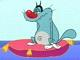# Math Is Fun Forum

Discussion about math, puzzles, games and fun.   Useful symbols: ÷ × ½ √ ∞ ≠ ≤ ≥ ≈ ⇒ ± ∈ Δ θ ∴ ∑ ∫  π  -¹ ² ³ °

You are not logged in.

## #1 2009-08-14 06:19:48

lashko
Member
Registered: 2009-08-13
Posts: 8

### Sum of n-th degrees

Hi everyone

Look, we know how to calculate ∑i , ∑(i^2) , ∑(i^3) when i is any positive integer from 1 to n and we can proove them by arithmetic sequence rules or induction, but, can we find a general formula for ∑(i^4)?

Last edited by lashko (2009-08-14 06:21:07)

Offline

## #2 2009-08-14 07:33:19

juriguen
MemberRegistered: 2009-07-05
Posts: 59

### Re: Sum of n-th degrees

Hi!

Apparently, according to http://en.wikipedia.org/wiki/Sum

Moreover:

where Bk is the kth Berboulli number.

And in http://en.wikipedia.org/wiki/Bernoulli_number

Jose

Last edited by juriguen (2009-08-14 07:40:48)

Make everything as simple as possible, but not simpler. -- Albert Einstein

Offline

## #3 2009-08-14 13:10:17

bobbym
bumpkinFrom: Bumpkinland
Registered: 2009-04-12
Posts: 109,606

### Re: Sum of n-th degrees

Hi lashko;

The usual method to sum series like that is to convert k^4 to the so
called factorial powers and then use the methods of the
summation calculus. It's pretty boring.

A cuter method is by interpolation.
Since you know that the sum is going to be a fifth order polynomial,
you want to fit for a,b,c,d,e,f of

Now fire up your handheld, your package or take it to wolfram alpha. There are other websites that solve simultaneous sets of equations. You will get

a= 0
b= -(1/30)
c= 0
d= 1/3
e= 1/2
f = 1/5

So

Of course you would use induction to verify that the above sum is correct.

Last edited by bobbym (2009-08-14 17:09:48)

In mathematics, you don't understand things. You just get used to them.
If it ain't broke, fix it until it is.
Always satisfy the Prime Directive of getting the right answer above all else.

Offline

## #4 2009-08-14 14:06:48

integer
Member
Registered: 2008-02-21
Posts: 79

### Re: Sum of n-th degrees

lashko wrote:

Hi everyone

Look, we know how to calculate ∑i , ∑(i^2) , ∑(i^3) when i is any positive integer from 1 to n and we can proove them by arithmetic sequence rules or induction, but, can we find a general formula for ∑(i^4)?

Look up "Faulhaber's Formula"

http://mathworld.wolfram.com/FaulhabersFormula.html

There are formulas on the net for the exponent value up to 250 (or so).  The LENGTH of the intermediate calc's get's LONG.

I believe Faulhaber (in the year 1630 or so) was able to calculate developed the formula for the exponent up to 17.

Bernoulli polynomials are helpful in computing exponential sums.

Offline

## #5 2009-08-14 21:40:17

Kurre
MemberRegistered: 2006-07-18
Posts: 280

### Re: Sum of n-th degrees

Look at mathsyperson proof of the sum of squares:
http://www.mathisfunforum.com/viewtopic.php?pid=18619#p18619
This proof can be generalized so, if you know the formula for all sums with powers up to k, we can express the sum to the power k+1 in the other sums.

Offline

## #6 2009-08-14 21:49:54

bobbym
bumpkinFrom: Bumpkinland
Registered: 2009-04-12
Posts: 109,606

### Re: Sum of n-th degrees

Hi lashko;

Another and perhaps the easiest way is to use the Euler MacLaurin summation formula. This formula relates sums, derivatives and integrals: I have truncated the formula since we don't require the fifth or greater derivatives. When f(x) is a polynomial the formula will give exact answers.

Where f(x) = x^4

After doing the integral and plugging in:

After simplification you get the same answer as post #3:

Last edited by bobbym (2009-08-15 09:44:34)

In mathematics, you don't understand things. You just get used to them.
If it ain't broke, fix it until it is.
Always satisfy the Prime Directive of getting the right answer above all else.

Offline